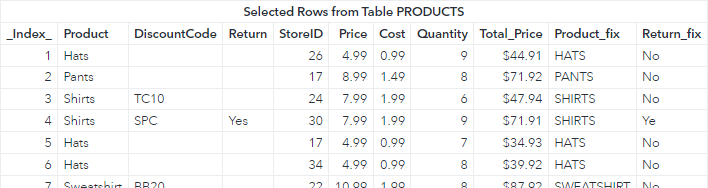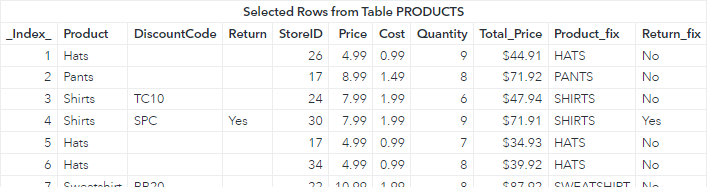Welcome back to my SAS Users blog series CAS Action! - a series on fundamentals. This post builds upon CAS-Action! Create Columns in CAS Tables - Part 1 by showing how to add formats and modify the length of computed columns.

I'll start by building off the following code where I create three new columns in the PRODUCTS table:

```proc cas; source createColumns; Total_Price = Price * Quantity; Product_fix = upcase(Product); if Return = "" then Return_fix = "No"; else Return_fix = "Yes"; endsource;   productsTbl = {name = 'products', caslib = 'casuser', computedVarsProgram = createColumns };   table.fetch / table = productsTbl; quit;```

Notice in the results the Total_Price column does not have a format, and the Return_fix column truncates the value Yes. Let's fix these issues.

## Add Formats to a Computed Column

First, I'll add a format to the Total_Price computed column. To add formats to a computed column use the computedVars sub-parameter. ComputedVars enables you to specify column metadata (labels and formats) for computed columns. The parameter expects a list of dictionaries, even if one column is specified.

For example, in the computedVars sub-parameter I'll add list with a single dictionary that specifies the name of a column Total_Price and apply the SAS dollar format. Next, I'll execute the fetch action to preview the table.

```proc cas; source createColumns; Total_Price = Price * Quantity; Product_fix = upcase(Product); if Return = "" then Return_fix = "No"; else Return_fix = "Yes"; endsource;   productsTbl = {name = 'products', caslib = 'casuser', computedVars = { {name = 'Total_Price', format = 'dollar16.2'} }, computedVarsProgram = createColumns };   table.fetch / table = productsTbl; quit;```

Notice in the results above the format was applied to the Total_Price column. However, now the additional computed columns are not displayed in the output. To fix this issue, add all of the computed columns to the computedVars sub-parameter as a dictionary with the name key, even if you do not modify the column metadata.

In the code below, the Product_fix and Return_fix columns were added to the list in the computedVars sub-parameter.

```proc cas; source createColumns; Total_Price = Price * Quantity; Product_fix = upcase(Product); if Return = "" then Return_fix = "No"; else Return_fix = "Yes"; endsource;   productsTbl = {name = 'products', caslib = 'casuser', computedVars = { {name = 'Total_Price', format = 'dollar16.2'}, {name = 'Product_fix'}, {name = 'Return_fix'} }, computedVarsProgram = createColumns };   table.fetch / table = productsTbl; quit;```

And the results:

##Notice in the results that adding each computed column in the computedVars sub-parameter fixed the issue and the computed columns are shown.

However, we still have one more issue. Look at the truncated values in the Return_fix column. How can we fix that?

## Modify Computed Column Lengths

When using an IF/THEN/ELSE statement to create a new column in SAS, it sets the column width by using the length of the first assignment statement. In this example, the first assignment statement sets the value of the Return_fix column to No, so the column length is set to 2.

To modify the length of the computed column add a SAS LENGTH statement in the computedVarsProgram parameter prior to the first assignment statement. Here I'll set the Return_fix column to length of 3.

```proc cas; source createColumns; Total_Price = Price * Quantity; Product_fix = upcase(Product);   length Return_fix varchar(3); if Return = "" then Return_fix = "No"; else Return_fix = "Yes"; endsource;   productsTbl = {name = 'products', caslib = 'casuser', computedVars = { {name = 'Total_Price', format = 'dollar16.2'}, {name = 'Product_fix'}, {name = 'Return_fix'} }, computedVarsProgram = createColumns };   table.fetch / table = productsTbl; quit;```

And the results:

##In the results the truncation issues in the Return_fix column are corrected.

## Summary

In summary, using the computedVars sub parameter allows you to add formats to the computed columns, and the computedVarsProgram parameter enables you to set the column lengths. A few key points:

• When using the computedVars sub-parameter, all computed columns from the computedVarsProgram sub-parameter must be added. Otherwise, the computed columns are not returned.
• The computedVars sub-parameter takes a list of dictionaries as it's value.